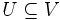# Locally contractible space

Jump to: navigation, search
This article defines a property of topological spaces: a property that can be evaluated to true/false for any topological space|View a complete list of properties of topological spaces
This is a variation of contractibility. View other variations of contractibility

## Definition

### Symbol-free definition

A topological space$X$ is said to be locally contractible if it satisfies the following equivalent conditions:

1. It has a basis of open subsets each of which is a contractible space under the subspace topology.
2. For every$x \in X$ and every open subset$V \ni x$ of$X$, there exists an open subset$U \ni x$ such that$U \subseteq V$ and$U$ is a contractible space in the subspace topology from$V$.